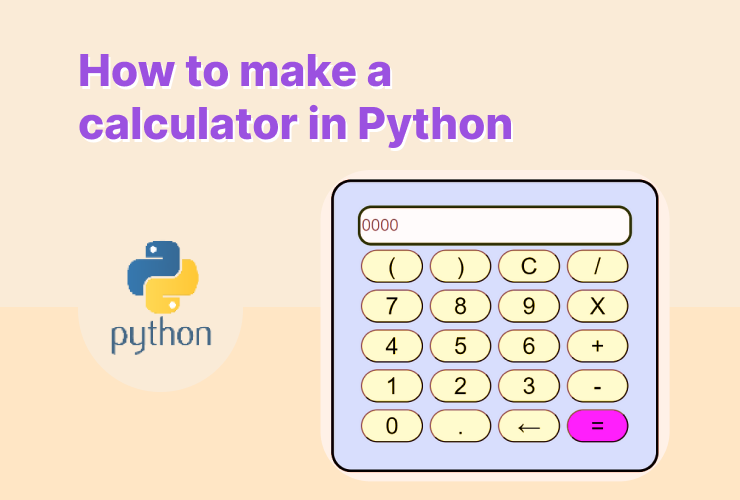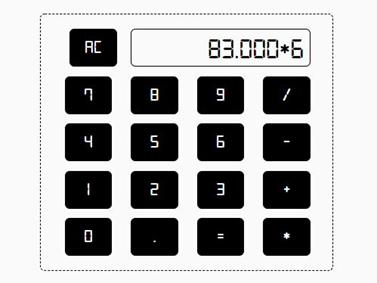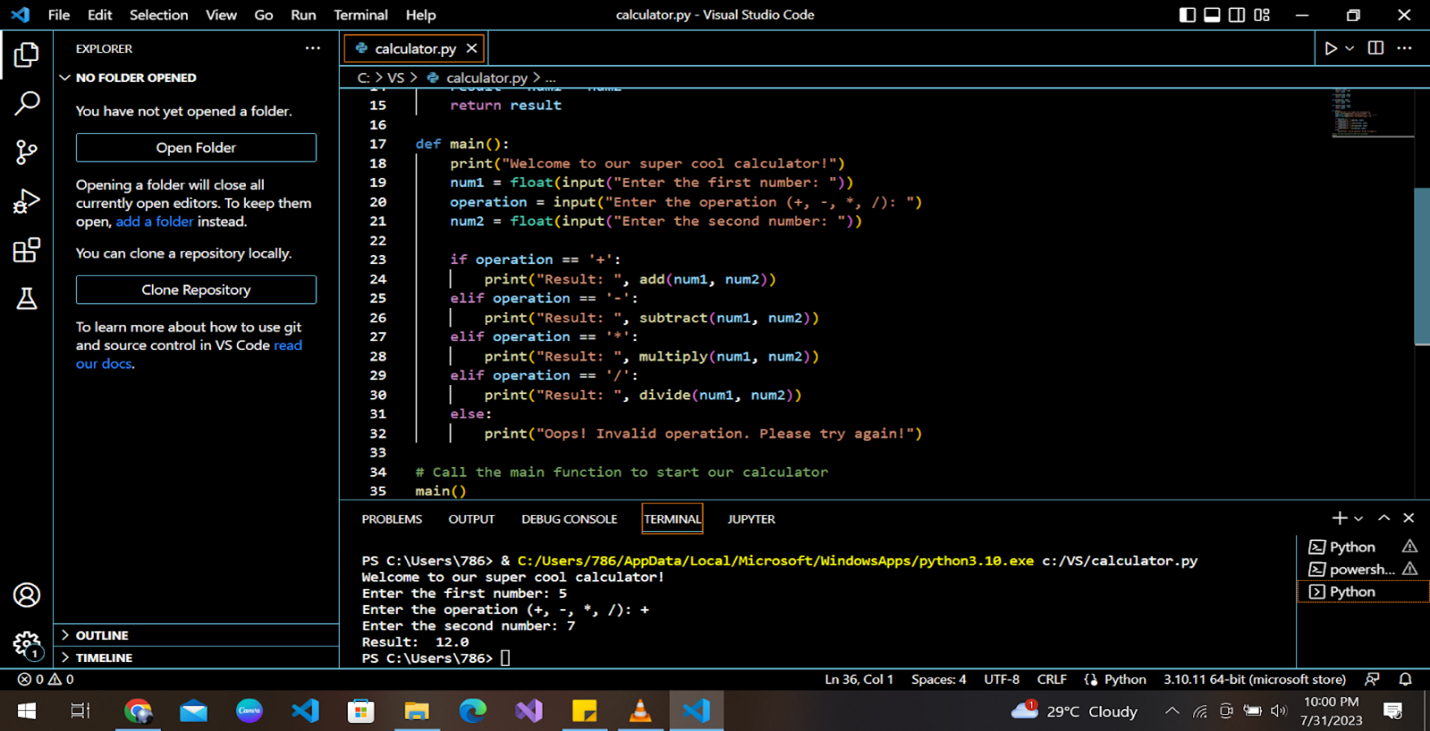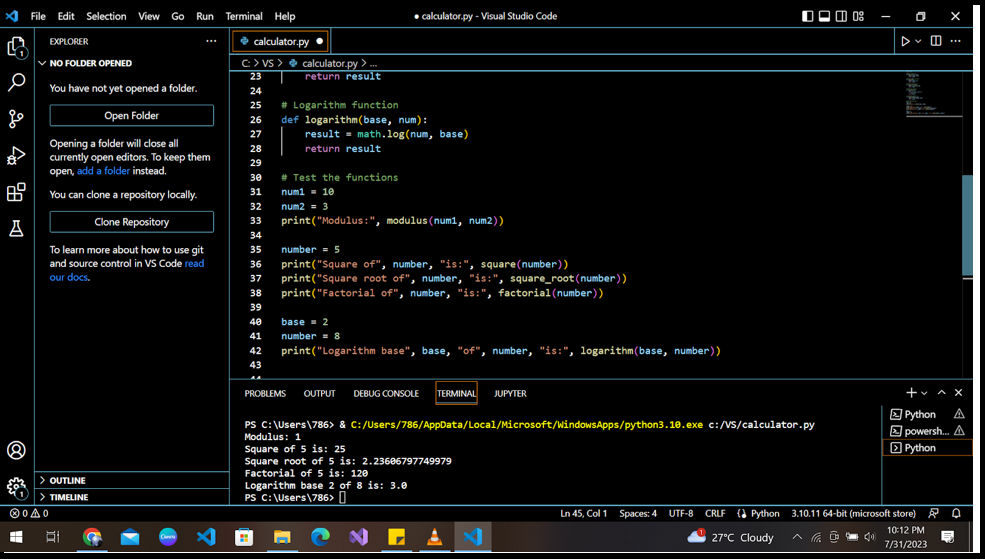# How to Make a Calculator in Python

Shreya on August 26, 2023## Introduction

Are you ready to create something super cool and helpful? Today, we’ll learn how to build a calculator using Python! We’ll take small steps, use easy language, and write sample code to make things clear and concise. So, please put on your coding hats, and let’s get started!

## Set-up

Do you know how we use calculators to add numbers, subtract, multiply, and even divide them? We’ll create a similar digital calculator that can do all these calculations for us!To build your calculator, you will need Python installed on your computer. You can get it for free from the Python website (python.org) and install the latest version easily. You can visit their website for a detailed guide on how to install Python.

Open your code editor, and let’s create a new Python file. We’ll call it “calculator.py”. Ready? Great! Now, let’s start coding!

## Functions in Python

Functions in Python are like little helpers that perform specific tasks for us. They are blocks of code that you can define once and then call (use) whenever you need them to perform a particular action.

Think of a function as a mini-program that takes some inputs (if needed) performs a task, and gives you an output (if specified). For our calculator, we will define different functions for addition, subtraction, division, and multiplication separately.

Our code should be able to input 2 or more numbers, add them, and give the output. Here is the code to achieve this:

```def add_numbers(num1, num2):
result = num1 + num2
return result```

We use the “def” keyword to define a function in Python. The common convention to define and call a function is:

```def function_name(input parameter1, ...)
#some functional code
return variable_name

#calling a function
function_name(arg1, …)```

## Subtraction

What changes do you think we need to make in the addition function to convert it into a subtraction function? You guessed it right! The name of the function and the addition sign.

```def subtract(num1, num2):
result = num1 - num2
return result```

## Division

Imagine I give you an 8-sliced pizza that you need to share with your friend. How many slices will each of you get? Can you solve this problem using your Python calculator?

```def divide(num1, num2):
result = num1 / num2
return result```

Well, yes! If you put the value of num1 as 8, and num2 as 2, then you can easily calculate the number of slices each one of you would get.

## Multiplication

Repeated addition can be a method to achieve multiplication, but how would you write multiplication code for constants? Well, it’s pretty easy.

```def multiply(num1, num2):
result = num1 * num2
return result```

## Putting it all together

Now that we have our functions ready let’s bring them together and create the main part of our calculator. We’ll ask the user for input and perform the desired calculation using our magical functions!

```def main():
print("Welcome to our python calculator!")
num1 = float(input("Enter the first number: "))
operation = input("Enter the operation (+, -, *, /): ")
num2 = float(input("Enter the second number: "))

if operation == '+':
elif operation == '-':
print("Result: ", subtract(num1, num2))
elif operation == '*':
print("Result: ", multiply(num1, num2))
elif operation == '/':
print("Result: ", divide(num1, num2))
else:
print("Oops! Invalid operation. Please try again!")

# Call the main function to start our calculator
main()```

Now, let’s execute our calculator. It may look something like this:We all know that current calculators are so advanced, and they solve complex mathematical problems in seconds. You can customize your Python calculator to implement all these functionalities too.

Let’s write simple functions for modulus, square number, square root, factorial, and logarithm.

import math

# Modulus function
def modulus(num1, num2):
result = num1 % num2
return result

```# Square number function
def square(num):
result = num * num
return result

# Square root function
def square_root(num):
result = math.sqrt(num)
return result

# Factorial function
def factorial(num):
result = 1
for i in range(1, num + 1):
result *= i
return result

# Logarithm function
def logarithm(base, num):
result = math.log(num, base)
return result```

Now let’s see if the functions that we wrote are working perfectly or not.## Conclusion

You did it! You’ve learned how to make a super cool calculator in Python – a powerful tool that can perform addition, subtraction, and so much more with ease. You’ve explored the magic of functions, how to take user input, and how to bring it all together to create a fantastic program.

Keep experimenting with Python, and who knows what else you’ll be able to create in the future? Coding is an exciting adventure, and the possibilities are endless.

You can take the time to master the fundamentals of Python by taking our awesome Python courses. Let’s learn and program together!